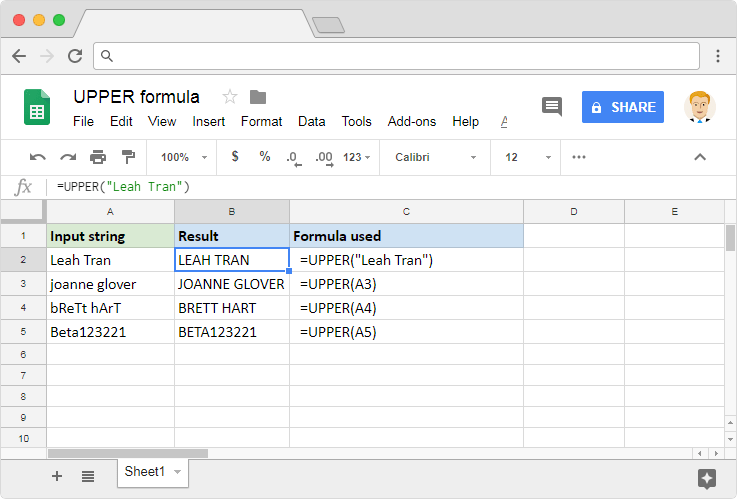# How to use the UPPER formula in Google SheetsNumbers are what comes to our mind when we think of spreadsheets applications. That is no surprise because they simplify a lot of calculations for us. Thankfully, their support is beyond just the numbers. We also have solid help in the form of many text-based formulas. Using them, we can manipulate the strings in many ways depending on our requirements. The UPPER formula in Google Sheets is one such formula. It helps us to convert the text from lowercase to uppercase.

### Syntax

UPPER(text)

• text – is the input string that we need uppercase conversion for.

### Usage: UPPER formula in Google Sheets

We may have observed this is probably the simplest of syntaxes any Google Sheets formula can have. Although it is very straightforward as to what it does, let us look at a few examples to ensure we understand its behavior. Consider the snapshot below.In the first example, the formula converted the instances of lowercase letters, other than G and R, are into uppercase letters. Here the input is a direct text string, enclosed within a pair of double quotes. It may not always be feasible to manually type in the input strings. So, to save us from that effort, the LOWER formula also accepts references to cells that host these text values. Which is why we used cell references in the next three examples.

Regardless of the position of a lowercase character within the string, the formula converts every such instance to an uppercase character. It could be that all of the text is in lowercase (example 2), or in uppercase. Or it could also be that the characters in the input string are randomly lowercase and uppercase (example 3). It doesn’t matter how many lowercase characters there are within the input string. The result the formula always generates an output in which every alphabet is in uppercase. That is true even in the case of alphanumeric strings (example 4).